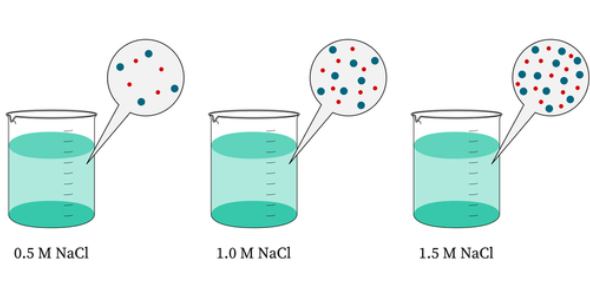# Molarity Practice Quiz

10 Questions | Attempts: 13280
ShareSettingsAre you ready to take this molarity practice quiz that we have here for you? If so, take the quiz then! The concentration of a solution can be calculated even before it is formed by the use of the number of moles they have. Do you have an upcoming chemistry exam where you need to study morality? This quiz will help you practice molarities calculations. Give it a try, and all the best! Let's go for it!

• 1.
Sea water contains roughly 28.0 g of NaCl per liter. What is the molarity of sodium chloride in seawater?
• A.

0.479M

• B.

0.488M

• C.

0.499M

• D.

0.610M

• 2.
What is the molarity of 5.30 g of Na2CO3 dissolved in 400.0 mL solution?
• A.

0.105M

• B.

0.125M

• C.

0.25M

• D.

0.225M

• 3.
What is the molarity of 5.00 g of NaOH in 750.0 mL of solution?
• A.

0.15M

• B.

0.167M

• C.

0.172M

• D.

0.184M

• 4.
The molarity of 10.00 g of NaOH in 1900.0 mL of solution is
• A.

0.152M

• B.

0.178M

• C.

0.252M

• D.

None of these

• 5.
What is the molarity of a solution made by dissolving 20.0 g of H3PO4 in 50.0 mL of solution?
• A.

3.08M

• B.

4.08M

• C.

5.08M

• D.

6.08M

• 6.
0.450 moles of NaCl are dissolved in 95.0 mL of water. Calculate the molarity of the NaCl solution.
• A.

4.7M

• B.

4.8M

• C.

4.9M

• D.

5.0M

• 7.
15 g of NaCl occupy a volume of 75 mL. What is the molarity of the solution?
• A.

3.4M

• B.

3.6M

• C.

3.8M

• D.

4.0M

• 8.
45.0 g of Ca(NO3)2 was used to create a 1.3 M solution. What is the volume of the solution?
• A.

115ml

• B.

190ml

• C.

210ml

• D.

250ml

• 9.
What is the molarity of sodium chloride in river water?
• A.

0.455M

• B.

0.488M

• C.

0.510M

• D.

None of these

• 10.
How many moles of Ca(NO3)2 are there in 75 mL of 0.25 M solution?.
• A.

0.0188mol

• B.

0.02mol

• C.

0.0321mol

• D.

0.044mol

## Related TopicsBack to top
×

Wait!
Here's an interesting quiz for you.